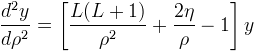Computes the regular and irregular Coulomb wave functions and their derivatives.

Namespace:  Meta.Numerics.Functions
Assembly:  Meta.Numerics (in Meta.Numerics.dll) Version: 4.1.4Syntax
```public static SolutionPair Coulomb(
int L,
double eta,
double rho
)```

#### Parameters

L
Type: SystemInt32
The angular momentum number, which must be non-negative.
eta
Type: SystemDouble
The charge parameter, which can be positive or negative.
rho
Type: SystemDouble
The radial distance parameter, which must be non-negative.

#### Return Value

Type: SolutionPair
The values of F, F', G, and G' for the given parameters.Exceptions
ExceptionCondition
ArgumentOutOfRangeExceptionL or rho is negative.Remarks

The Coulomb wave functions are the radial wave functions of a non-relativistic particle in a Coulomb potential.

They satisfy the differential equation:A repulsive potential is represented by η > 0, an attractive potential by η < 0.

F is oscillatory in the region beyond the classical turning point. In the quantum tunneling region inside the classical turning point, F is exponentially suppressed and vanishes at the origin, while G grows exponentially and diverges at the origin.

Many numerical libraries compute Coulomb wave functions in the quantum tunneling region using a WKB approximation, which accurately determine only the first few decimal digits; our library computes Coulomb wave functions even in this computationally difficult region to nearly full precision -- all but the last 4-5 decimal digits can be trusted.

The irregular Coulomb wave functions GL(η,ρ) are the complementary independent solutions of the same differential equation.See Also